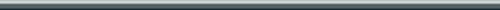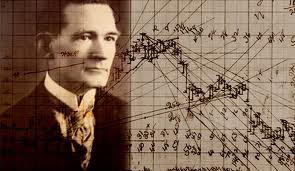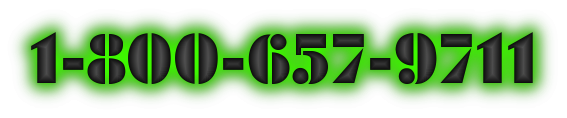Always study the financial markets extensively, including time-cycles and master-time-factor to trade with success starting today which can be the date you may change your trend from a losing trader to get in the winners circle by becoming a winning trader!

To arrive at the strongest and most important points within a Gann Square, I divide it into 2 equal parts by drawing a horizontal and a vertical line. Note angle marked "A" which divides each of the smaller squares into 2 equal parts and runs from 0 to 28 diagonally. This is a diagonal line moving on a 45° angle and thus it divides the large square into 2 equal parts. Then, note the angle "B" at 14, running horizontally across chart. This divides the square into 2 equal parts. Note angle "C" which is a vertical line running up from 14, which is ½ of 28.

This extremely significant 45-Degree Gann Angle (always the single most important gravity center) crosses at the center or ½-point at 14 where the other geometric angles cross-over each other, thus dividing the square into 2 equal parts. Then, note angle "D" which forms another 45° angle moving from the N.W. corner to the S.E. corner, crossing 14 at the exact ½-point. You can visualize by this if we draw the first line thru the square center, we divide it into 2 equal parts. When we draw the lines from the other directions, we divide it into 4 equal parts. Then, by drawing the 2 lines from each corner, we divide the square into 8 equal parts and produce 8 triangles. We use 28 because 28¢ was the lowest price cash-market Wheat has ever sold at.

As you study the square, it should be relatively easy for you to tell with your eyes where the strongest support point or resistance points are located. It is at the (gravity) center where all the angles cross. Four angles cross at this point so, naturally, this would be a stronger support point than a place where only one angle crosses.

We can also divide each one of these smaller squares into 4 or 8 equal parts by drawing angles in the same way. Later, when I give you the rules and examples, I will explain how to square the range of a stock or commodity that is, the difference between extreme low and extreme high market price, or the difference between any low point and any high point, and also how to square the bottom price.

## Pattern Chart For Drawing Gann Geometrical Angles

The Square Of 90, or the Pattern Chart, shows all the measured angles that are important to use in determining the position of an option. These angles are as follows: 3¾, 7½, 15, 18¾, 26¼, 30, 33¾, 37½, 45, 52½, 56¼, 60, 63¾, 71¼, 75, 82½, 86¼ and 90°.

It's not necessary to measure these angles with a protractor. All you have to do to get the angles correct is count the spaces on the chart paper, using 8 x 8 to the inch, and draw the lines or angles accordingly. On the square of 90 (which you will understand with instructions), note how equal angles drawn from the top and from the bottom prove themselves by the point at which they cross.

Example: The angles of 8x1 from 90 down both cross at 45, 5⅝-points over from 0 counting to the right. Then, the angle of 4x1 from 0 and the angle of 4x1 down from 90, you will notice, cross at 11¼ on 45, equal distance from the other angle and twice the measure. The reason these angles prove this way is because the 45° angle, or 45-points or degrees from 0 to 45, is ½ of go. Therefore, parallel angles, beginning at 0 going up and at 90 coming down, must cross on a 45° angle or at the gravity center.

## How To Draw Angles From A Low Point Recorded On Grain Options

An example marked "Form #2" shows you the most important angles to use when Grain is working higher and advancing.

First Important Geometrical Angle: 45-degree angle, a.k.a. 1X1 angle

The first and always most important geometric angle to draw is a 45° angle or a moving-trend-line moving up 1¢ per day, 1¢ per week or 1¢ per month. This is a 45° angle because it divides the space and time periods into 2 equal parts. As long as the market or an option stays above the 45° angle, it is in a strong position and indicates higher prices. You can buy every time it rests on the 45° angle with a STOP-LOSS order 1¢, 2¢ or 3¢ under the 45° angle, but remember the rule: Never use a STOP-LOSS order more than 3¢ away. Unless Grain options are near the low levels, or just starting in a bull market or selling at very low prices, I always use a STOP-LOSS order 1¢ under the 45° angle. If this angle is broken by 1¢, you will usually find that the trend has changed, at least temporarily, and the option will go lower.

A relatively simple way to calculate with good accuracy how to put on this 45° angle, is for example: If the time=frame is say 28-days, 28-weeks or 28 months from the price where the option was bottom, then the angle of 45° must be 28¢ up from the bottom and would cross at 28. This is one of the easiest angles to put on and one of the simplest to learn. You can beat the market by trading against the 45° angle alone, if you stick to the rule: Wait to buy an option on the 45° angle or wait to sell the market against the 45° angle.

Second Important Angle: 2x1

This is the 2x1 angle, or the moving trend line, which moves up at the rate of 2¢ per day, week or month. It divides the space between the 45° angle and the vertical angle into 2 equal parts and measures 63¾°. That is why it is the next strongest and most important angle.

As long as Grain holds above this angle, it's in a stronger position than when it is resting on a 45° angle because it is a more acute angle. When an option breaks under this angle of 2x1, or 2¢ for each time period, then it indicates that it will go lower and reach the 45°. Remember the rule of all angles: No matter what angle the option breaks under, it indicates a decline to the next major angle below it.

Third Most Important Angle: 4x1

Still stronger as long as an option holds above it is the angle, which moves up 4¢ per day, week or month. This angle is 4x1 or 4-points of space or price equals 1 period of time. It measures 75° and divides the space between the angle of 2x1 and the 90° angle into 2 equal parts. Any option that continues to advance 4¢ per day, 4¢ per week or 4¢ per month, and remains above this angle is in a very strong position as long as it stays above it, but when it breaks under, it indicates the next angle or next support point according to the position of the option on time and resistance levels.

Fourth Important Angle: 8x1

The angle of 8x1 or the one that moves up 8¢ per day, week or month measures 82½°. As long as the option can hold above this angle on daily, weekly or monthly charts, it is in the strongest possible position but when it reverses trend and declines below this angle, then it indicates a decline to the next important angle.

Fifth Important Angle: 16x1

It is possible to use an angle of 16x1, or 16¢ of price to 1 period of time, which measures 86¼°, but this angle is only used in fast, advancing markets like 1947-1948, when Grains are moving up or down 16¢ or more per week or month. There are very few options that will move up 16¢ per day, week or month, and very seldom.

You will note that with these four important angles we show the strong or bullish side of the market. All the time, by dividing the space with angles, we are getting the ½-point or the gravity center of time and price.

Sixth Important Angle: 3x1

Note the angle drawn in green marked "3x1" which moves up at the rate of 3¢ per day, week or month, measuring 71¼°. This angle is important at times, after markets have had a prolonged advance and are a long distance up from the bottom. It is an important angle to use on monthly and weekly charts.

These are the important angles you need to work with; as long as an option continues to advance and work up and stays above the angle of 45°, or the trend line of 1¢ per day, week or month.

While there are 360° in a circle, and angles can form at any of the 360-degrees, all of the important angles form between 0 and 90 because 90 is straight up and down and the most acute angle on which price can rise.

Example: The 45° angle divides the space from 0 to 90 in half. The angle of 135° is another angle of 45° because it is ½ of the next quadrant between 90 and 180. 225 and 315 in a circle are also 45° geometric angles. Therefore, all of the angles are valuable in determining the trend of Grain are found between 0 and 90°. When we divide 90° by 8, we get the most important angles to use, then divide it by 3 and we get 30 and 60° angles, which are important to use for time and resistance points, and support-levels too.

Here are some (but not all) of the key subjects covered in our highly recommended Gann Techniques Trading Course, which can effectively be used to trade stocks or commodities using amazing Gann techniques: Did William D. Gann use Astrology in his Trading? (the answer may surprise you), Square Chart Definition and Usage; Squaring Price and Time; Geometric Angles; Angle Concepts; Gaps on Charts; What Causes Chart Gaps; The Gann Square of Nine and other powerful Gann trading methodologies, plus more about How To Square Price and Time.This potentially highly profitable but low-cost Gann Secrets (explained) trading course offers every Gann trader (both new and experienced) the information needed to blend these powerful Gann trading techniques into commodities, futures & stock market trading and trade decisions, which can bring you consistent profits.

For traders who actively trade (or wish to learn more on how to trade) the financial, stocks or futures markets using Gann techniques, there are a lot of other issues outside the markets you should be following. But, I guess my bigger message is for those of you that aren’t in the futures markets, whether you trade them or not, the futures markets have a significant impact on what happens in the other financial markets, including forex, currencies, mutual funds, options and stocks.

#### Websites of InterestToday's Date and Time Copyright©All Rights Reserved | Privacy-Policy